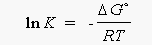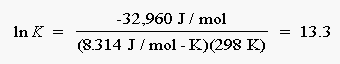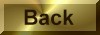Practice Problem 9

Use the value ofGo obtained in Practice Problem 7 to calculate the equilibrium constant for the following reaction at 25°C:

N2(g) + 3 H2(g)2 NH3(g)

Solution

Practice Problem 7 gave the following value forGo for this reaction at 25°C:Go = -32.96 kJ

Now we turn to the relationship betweenGo for a reaction and the equilibrium constant for the reaction:Go = - RT ln K

Solving for the natural log of the equilibrium constant gives the following equation:Substituting the known value ofGo, R, and T into this equation gives the following result:The equilibrium constant for this reaction at 25°C is therefore 6.0 x 105:

K = e13.3 = 6.0 x105

It is easy to make mistakes when handling the sign of the relationship betweenGo and K.  It is therefore a good idea to check the final answer to see whether it makes sense.Go for the reaction in this exercise is negative.  The reaction is therefore spontaneous, and the equilibrium should lie on the side of the products.  The equilibrium constant should therefore be much larger than 1, which it is.